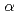※ 半角英数字
年 ～
年

# Spectrum prediction in X-ray fluorescence analysis using Bayesian estimation

## ベイズ推定法を用いた蛍光X線分析におけるスペクトル予測

It is important to reduce the measurement time in X-ray fluorescence (XRF) analysis. Micro XRF and confocal micro XRF analyses have been used to obtain elemental distribution. Because these techniques are performed in the scanning mode, shortening the measurement time per unit measurement point enables rapid determination of the elemental distributions. Therefore, we applied the Bayesian theorem to XRF analysis to estimate the accurate XRF net intensity in a short time. In the Bayesian formula, the posterior distribution is determined by the likelihood function and prior distribution. As the obtained posterior function is a probability distribution, the expected value in the function is used as the optimal value. By determining the optimal likelihood function and prior distribution, we consider that the XRF spectrum in a long-time measurement can be estimated by that in a short time. In this study, the Poisson distribution and the sum of the two exponential functions were employed as the likelihood function and prior distribution, respectively. To estimate the XRF spectrum using the Bayesian formula, a standard glass sample containing several metal elements was analyzed using a laboratory-made micro XRF instrument. The micro XRF measurements were performed at measurement times of 1, 3, 5, 7, 10, 20, 30, 60, 100, 180, and 3600 s, and then the net intensity of Zn Kobtained with and without the Bayesian estimation was compared. To obtain a net intensity of Zn Kclose to that in 3600 s, the measurement times with and without the Bayesian estimation were required to be 3 and 7 s, respectively. Thus, we significantly reduced the measurement time for an accurate XRF net intensity measurement by more than 50%.

Access Altmetrics : - Accesses :
• 登録番号 : AA20220424
• 抄録集掲載番号 : 51000055
• 論文投稿番号 : 26048

[CLARIVATE ANALYTICS], [WEB OF SCIENCE], [HIGHLY CITED PAPER & CUP LOGO] and [HOT PAPER & FIRE LOGO] are trademarks of Clarivate Analytics, and/or its affiliated company or companies, and used herein by permission and/or license.